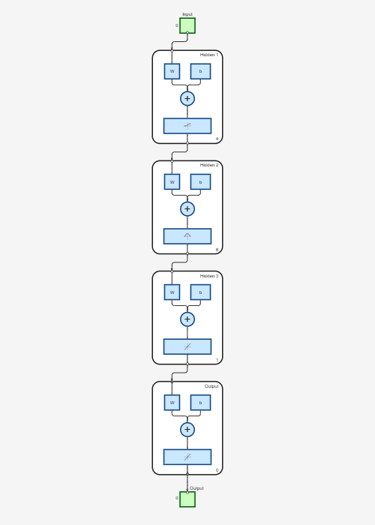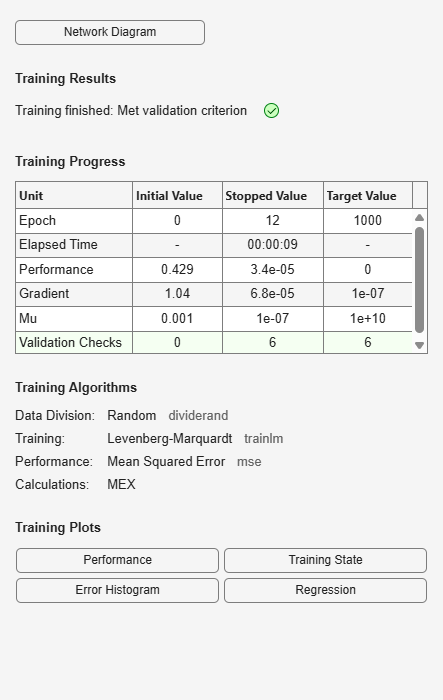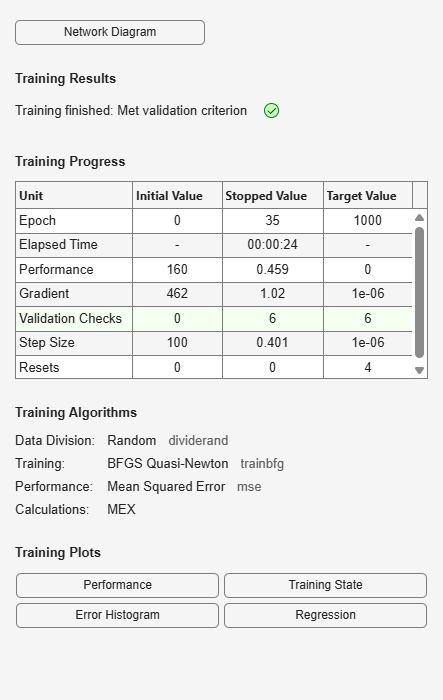Main Content

# neuralnet

Class representing neural network nonlinearity estimator for nonlinear ARX models

## Syntax

```net_estimator = neuralnet(Network) ```

## Description

`neuralnet` is the class that encapsulates the neural network nonlinearity estimator. A `neuralnet` object lets you use networks, created using Deep Learning Toolbox™ software, in nonlinear ARX models.

The neural network nonlinearity estimator defines a nonlinear function $y=F\left(x\right)$, where F is a multilayer feed-forward (static) neural network, as defined in the Deep Learning Toolbox software. y is a scalar and x is an `m`-dimensional row vector.

You create multi-layer feed-forward neural networks using Deep Learning Toolbox commands such as `feedforwardnet` (Deep Learning Toolbox), `cascadeforwardnet` (Deep Learning Toolbox) and `linearlayer` (Deep Learning Toolbox). When you create the network:

• Designate the input and output sizes to be unknown by leaving them at the default value of zero (recommended method). When estimating a nonlinear ARX model using the `nlarx` command, the software automatically determines the input-output sizes of the network.

• Initialize the sizes manually by setting input and output ranges to `m`-by-2 and 1-by-2 matrices, respectively, where `m` is the number of nonlinear ARX model regressors and the range values are minimum and maximum values of regressors and output data, respectively.

See Examples for more information.

Use `evaluate(net_estimator,x)` to compute the value of the function defined by the `neuralnet` object `net_estimator` at input value x. When used for nonlinear ARX model estimation, x represents the model regressors for the output for which the `neuralnet` object is assigned as the nonlinearity estimator.

You cannot use `neuralnet` when the `Focus` option in `nlarxOptions` is `'simulation'` because this nonlinearity estimator is considered to be nondifferentiable for estimation. Minimization of simulation error requires differentiable nonlinear functions.

## Construction

`net_estimator = neuralnet(Network)` creates a neural network nonlinearity estimator based on the feed-forward (static) network object `Network` created using Deep Learning Toolbox commands `feedforwardnet`, `cascadeforwardnet`, and `linearlayer`. `Network` must represent a static mapping between the inputs and output without I/O delays or feedback. The number of outputs of the network, if assigned, must be one. For a multiple-output nonlinear ARX models, create a separate `neuralnet` object for each output—that is, each estimator must represent a single-output network object.

## Properties

 `Network` Neural network object, typically created using the Deep Learning Toolbox commands `feedforwardnet`, `cascadeforwardnet`, and `linearlayer`.

After creating the object, you can use `get` or dot notation to access the object property values. For example:

```% List Network property value get(n) n.Network```

You can also use the `set` function to set the value of particular properties. For example:

`set(d, 'Network', net_obj)`
The first argument to `set` must be the name of a MATLAB® variable.

## Examples

collapse all

Create a neural network nonlinearity estimator using a feed-forward neural network with three hidden layers; transfer functions of types `logsig`, `radbas`,and `purelin`; and unknown input and output sizes.

Create a neural network.

```net = feedforwardnet([4 6 1]); net.layers{1}.transferFcn = 'logsig'; net.layers{2}.transferFcn = 'radbas'; net.layers{3}.transferFcn = 'purelin'; ```

View the network diagram.

```view(net) ```Create a neural network estimator.

```net_estimator = neuralnet(net); ```

Create a single-layer, cascade-forward network with unknown input and output sizes and use this network for nonlinear ARX model estimation.

Create a cascade-forward neural network with 20 neurons and unknown input-output sizes.

`net = cascadeforwardnet(20);`

Create a neural network nonlinearity estimator.

`net_estimator = neuralnet(net);`

Load estimation data.

```load twotankdata Data = iddata(y,u,0.2);```

Estimate nonlinear ARX model.

`Model = nlarx(Data,[2 2 1],net_estimator);`

Compare model response to measured output signal.

`compare(Data,Model)`Initialize the input-output sizes of a two-layer feed-forward neural network based on estimation data, and use this network for nonlinear ARX estimation.

Load estimation data.

```load iddata7 z7 z7 = z7(1:200);```

Create a template nonlinear ARX model with no nonlinearity.

`model = idnlarx([4 4 4 1 1],[]);`

This model has six regressors and is used to define the regressors. The range of regressor values for input-output data in `z7` is then used to set the input ranges in the neural network object, as shown in the next steps.

Obtain the model regressor values.

```R = getreg(model,'all',z7); R = R.Variables;```

Create a two-layer, feed-forward neural network and initialize the network input and output dimensions to 2 and 1, respectively. Use 5 neurons for first layer and 7 for second layer.

`net = feedforwardnet([5 7]);`

Determine input range.

`InputRange = [min(R);max(R)].';`

Initialize input dimensions of estimator.

`net.inputs{1}.range = InputRange;`

Determine output range.

`OutputRange = [min(z7.OutputData),max(z7.OutputData)];`

Initialize output dimensions of estimator and the choice of training function.

```net.outputs{net.outputConnect}.range = OutputRange; net.trainFcn = 'trainbfg';```

Create a neural network nonlinearity estimator.

`net_estimator = neuralnet(net);`

Specify the nonlinearity estimator in the model.

`model.Nonlinearity = net_estimator;`

Estimate the parameters of the network to minimize the prediction error between data and model. Estimate model.

`model = nlarx(z7,model);`

Compare model's predicted response to measured output signal.

`compare(z7(1:100),model,1)`## Algorithms

The `nlarx` command uses the `train` method of the `network` object, defined in the Deep Learning Toolbox software, to compute the network parameter values.

Introduced in R2007a

## SupportGet trial now